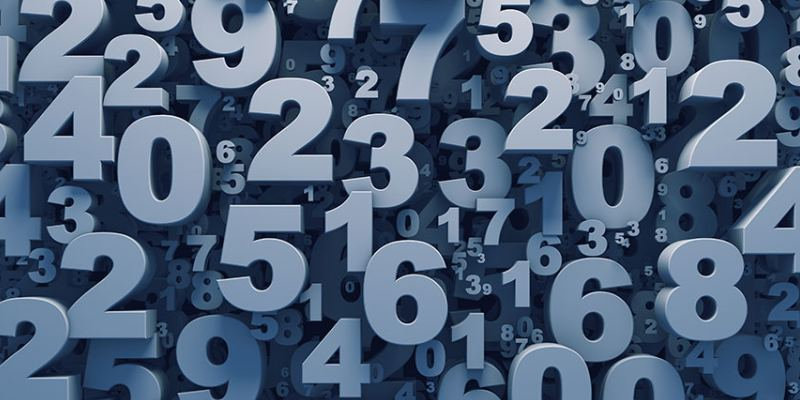# Ultimate Trivia Quiz On Numbers

Questions : 10 | Total Attempts: 418 | Recent Updated: 26-May-2020The number tells us how many units are there in a given quantity. A number denotes one or more units of the same kind of thing. For example two books, three tables, etc. There are different types of numbers like whole numbers, even numbers, odd numbers, primary numbers, composite numbers, consecutive numbers, rational numbers, irrational numbers. So Take this quiz and check your knowledge about numbers.

### Questions Excerpt

1. Which one of the following neither composite nor prime number?

A. 2

B. 0

C. 1

D. 5

2. Which one of the following is the even and prime number?

A. 0

B. 1

C. 2

D. 4

3. 0 is.....

A. Neither positive nor negative integer

B. Positive integer

C. Negative integer

D. None of these

4. Which one of the following is the smallest integer in positive integers?

A. +2

B. +1

C. +5

D. 0

5. ....... is the largest integer in negative integers.

A. 0

B. -7

C. -5

D. -1

6. The number which is only divisible by 1 or the number itself is called.......

A. Composite number

B. Consecutive numbers

C. Prime numbers

D. Rational number

7. When one number is the successor of the other is called....

A. Rational number

B. Consecutive number

C. Irrational number

D. Even number

8. The product of two prime numbers will be.....

A. Even number

B. Composite number

C. Whole number

D. Odd number

9. Face value of a digit in a number is the .....

A. Place of digit

B. Digit itself

C. Both of these

D. None of these

10. A number that cannot be expressed in the form of A/B is called.....

A. Rational number

B. Irrational number

C. Whole number

D. Natural number

## Quiz: Which Mathematician Are You?

Calling all lovers of numbers, equations, and mathematical wonders! Get ready to unlock the secrets of your mathematical identity with the captivating quiz, "Which Mathematician are You?" Embark on a thrilling journey through the annals of mathematic

## Accounting Basics Quiz and Test

Numbers are the only constant in our lives and are an essential part of our lives! No matter what we do, or where we are, you will see these numbers are the same. Even in our daily activities, numbers are more useful than we can imagine. Let us talk

## Orthographic Projection Quiz: How Much Do You Know Orthographic Projection?

Geometry, is undoubtedly a very interesting and captivating subject, as we all can see and must have studied. When it comes to science, we can all agree that there is no shortage of unexpected factors. We all studied these two concepts together in sc

## Decimals Quiz for 6th Grade Student

A decimal is a fragment that has been written in a particular way. Instead of writing 1/2, you could use the decimal 0.5 to symbolize the fraction, with the 0 withinside the one's vicinity and the 5 withinside the tenth's vicinity. Decimal derives fr

## Place Arrangement Trivia Quiz Questions and Answers

Place arrangement generally refers to the positioning of a person or object in a manner indicated by a set of information given. One has to understand the order of placement and then attempt questions by following the given information. It is a part

## Analogy Or Relationship Test Trivia Quiz Questions and Answers

In analogy tests, the relationship between two given words is established and applied to the other words. The type of relationship may vary, so while attempting such questions the first step is to identify the type of relationship, which can be any o

## Ultimate Trivia Quiz on Angle

In plane geometry, two rays that share a common endpoint and formed a figure is called angle and the two rays are called the sides of the angles. The endpoint of the rays called the vertex of the angle. The symbol angle is ∠. In general, angles are m

## Quiz: How Much You Know About Circle And Tangents?

A circle is a set of those points in a plane that are at a given constant from a given fixed point in the plane. The fixed point is called the center of the circle and the constant distance of every point on the circle from its center is called the r